# Variation of a set

A number characterizing the-dimensional content of a set in-dimensional Euclidean space. The zero variationof a closed bounded setis the number of components of this set.

In the simplest case of the plane, the linear variation of a set(i.e. the first-order variation of) is the integral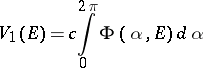of the function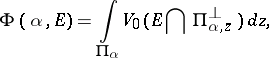where the integration is performed over the straight line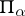passing through the coordinate origin,is the angle formed bywith a given axis and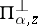is the straight line normal towhich intersects it at the point. The normalizing constantis so chosen that the variation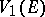of an intervalis equal to its length. For sufficiently simple sets, e.g. for rectifiable curves, the variation of the set is equal to the length of the curve. For a closed domainwith a rectifiable boundaryits linear variationis equal to one-half the length of. The second variation of(i.e. the second-order variation of) is the two-dimensional measure of, and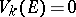if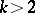.

In-dimensional Euclidean space the variationof order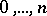, of a bounded closed setis the integral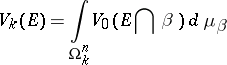of the zero variation of the intersection ofwith an-dimensional planein the spaceof all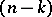-dimensional planes ofwith respect to the Haar measure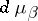; normalized so that the-dimensional unit cube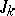has variation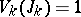.

The variation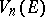is identical with the-dimensional Lebesgue measure of the set. For convex bodies the (suitably normalized) set variations are identical with Minkowski's mixed volumes (cf. Mixed-volume theory) .

### Properties of the variations of a set.

1) The variationsfor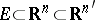calculated for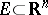and for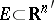have the same value.

2) The variations of a set can be inductively expressed by the formula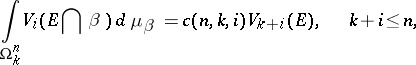where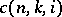is the normalization constant.

3)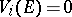implies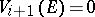.

4) In a certain sense, the variations of a set are not dependent, i.e. for any sequence of numbers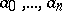, where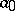is a positive integer,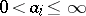(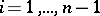),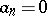, it is possible to construct a setfor which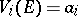,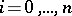.

5) If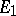and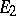do not intersect,. In the general case,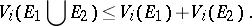Forthe variations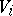are not monotone, i.e. it can happen for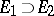that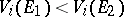.

6) The variations of a set are semi-continuous, i.e. if a sequence of closed bounded sets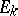converges (in the sense of deviation in metric) to a set, thenand if, in addition, the sums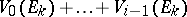are uniformly bounded, then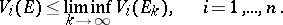7) The variation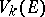becomes identical with the-dimensional Hausdorff measure ifand if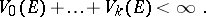These conditions are met, for example, by twice-differentiable manifolds.

The concept of the variation of a set arose in the context of solutions of the Cauchy–Riemann system, and its ultimate formulation is due to A.G. Vitushkin. The set variations proved to be a useful tool in solving certain problems in analysis, in particular that of superposition of functions of several variables , and also in approximation problems .